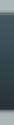Cockos Incorporated ForumsCollection of Sigmoid functions
 Register Track Bugs/Feature Requests Search Today's Posts Mark Forums Read11-02-2019, 03:21 AM #1 solarfall Human being with feelings   Join Date: Sep 2013 Posts: 42Collection of Sigmoid functions Hello, for all programmers here's a more complete collection of sigmoid functions for your waveshaping duties. https://www.desmos.com/calculator/d1n16t6xtp There are symmetrical and asymetrical waveshapers. Feel free to use them.11-02-2019, 06:11 AM #2 sai'ke Human being with feelings   Join Date: Aug 2009 Location: NL Posts: 665So many saturatorsI'm curious, is there a reason you chose to scale the output and arguments in this particular manner? For instance, afaik, ( e^x-e^(-x) ) / ( e^x+e^(-x) ) is just tanh(x), but then tanh(3x)/2 also appears in the list. Would be interesting to see the spectrum of each of these when applied to various signals. Have you listened to the effect of each of them? Which one is your favourite?__________________ [Tracker Plugin: Thread|Github|Reapack] | [Routing Plugin: Thread|Reapack] | [Filther: Thread|Github|Reapack] | [More JSFX: Thread|Reapack]11-02-2019, 06:48 AM #3 solarfall Human being with feelings   Join Date: Sep 2013 Posts: 42Hey Sai'ke, i actually listened to almost all of them. They all have slight different sounds. I'll implement a simple jsfx so you can listen to them.11-02-2019, 07:30 AM #4 solarfall Human being with feelings   Join Date: Sep 2013 Posts: 42Testing...last one crashed Reaper!! Removed...11-02-2019, 07:41 AM #5 solarfall Human being with feelings   Join Date: Sep 2013 Posts: 42Ok, tested it. Here's the code: Code: ```desc:Sigmoid Waveshaper slider1:0.5<0,1>Input: slider2:0<0,1,{0 (Lin),1 (Sym),2 (Sym),3 (Sym),4 (Sym),5 (Sym),6 (Sym),7 (Sym),8 (Sym),9 (Sym),10 (Sym),11 (Sym),12 (Sym),13 (Sym),14 (Sym),15 (Sym),16 (Asym),17 (Asym),18 (Asym),19 (Asym)}>Shape: slider3:0.5<0,1>Output: @init function tanh(x) ( y=(pow(\$e,2*x)-1)/(pow(\$e,2*x)+1); y; ); function ws0() ( spl0=spl0; spl1=spl1; ); function ws1() ( spl0=sin(spl0); spl1=sin(spl1); ); function ws2() ( spl0=atan(spl0); spl1=atan(spl1); ); function ws3() ( spl0=sin(atan(spl0)); spl1=sin(atan(spl1)); ); function ws4() ( spl0=sin(tan(spl1))*cos(atan(0.5*spl1)); spl1=sin(tan(spl1))*cos(atan(0.5*spl1)); ); function ws5() ( spl0=(pow(\$e,spl0)-pow(\$e,-spl0))/(pow(\$e,spl0)+pow(\$e,-spl0)); spl1=(pow(\$e,spl1)-pow(\$e,-spl1))/(pow(\$e,spl1)+pow(\$e,-spl1)); ); function ws6() ( spl0=0.7*tan(sin(1.4*spl0))*cos(0.7*spl0); spl1=0.7*tan(sin(1.4*spl1))*cos(0.7*spl1); ); function ws7() ( spl0=spl0/(1+abs(0.4*pow(spl0,3))); spl1=spl1/(1+abs(0.4*pow(spl1,3))); ); function ws8() ( spl0=sin(spl0)-(tan(spl0)/(4+pow(atan(spl0),2))); spl1=sin(spl1)-(tan(spl1)/(4+pow(atan(spl1),2))); ); function ws9() ( spl0=atan(1.4*pow(spl0,2))/(1.4*spl0); spl1=atan(1.4*pow(spl1,2))/(1.4*spl1); ); function ws10() ( spl0=(sin(2.5*spl0)+pow(x,3))/3; spl1=(sin(2.5*spl1)+pow(x,3))/3; ); function ws11() ( spl0=pow(spl0,3)/(pow(spl0,4)+pow(spl0,2)); spl1=pow(spl1,3)/(pow(spl1,4)+pow(spl1,2)); ); function ws12() ( spl0=(spl0+sin(2*spl0))/3.5; spl1=(spl1+sin(2*spl1))/3.5; ); function ws13() ( spl0=tanh(3*spl0)/2; spl1=tanh(3*spl1)/2; ); function ws14() ( spl0=atan(3*spl0)/2; spl1=atan(3*spl1)/2; ); function ws15() ( spl0=(2*spl0)/(1+abs(5*spl0)); spl1=(2*spl1)/(1+abs(5*spl1)); ); function ws16() ( spl0=sin(pow(1.1,tan(spl0))*spl0); spl1=sin(pow(1.1,tan(spl1))*spl1); ); function ws17() ( spl0=sin(spl0*sqrt(pow(2,spl0))); spl1=sin(spl1*sqrt(pow(2,spl1))); ); function ws18() ( spl0=(cos(spl0)/3)+sin(spl0)-0.33; spl1=(cos(spl1)/3)+sin(spl1)-0.33; ); function ws19() ( spl0=(1+1.6*spl0)/(1.6+pow(spl0,2))-0.6; spl1=(1+1.6*spl1)/(1.6+pow(spl1,2))-0.6; ); @slider @block @sample spl0 *= slider1*2; spl1 *= slider1*2; slider2 == 0 ? ws0(); slider2 == 1 ? ws1(); slider2 == 2 ? ws2(); slider2 == 3 ? ws3(); slider2 == 4 ? ws4(); slider2 == 5 ? ws5(); slider2 == 6 ? ws6(); slider2 == 7 ? ws7(); slider2 == 8 ? ws8(); slider2 == 9 ? ws9(); slider2 == 10 ? ws10(); slider2 == 11 ? ws11(); slider2 == 12 ? ws12(); slider2 == 13 ? ws13(); slider2 == 14 ? ws14(); slider2 == 15 ? ws15(); slider2 == 16 ? ws16(); slider2 == 17 ? ws17(); slider2 == 18 ? ws18(); slider2 == 19 ? ws19(); spl0 *= slider3*2; spl1 *= slider3*2;``` You can see the added harmonics with a tone generator and a spectrum analyzer. I used SPAN that works great.11-03-2019, 12:24 AM #6 SaulT Human being with feelings   Join Date: Oct 2013 Location: Seattle, WA Posts: 768Just thinking... room for customization here. For example, the second to last could be changed to (1+ax)/(a+x^2)-1/a I mention this because higher values of a make a more linear function, but specifically that a=2 makes a monotonic function so it isn’t folding over near 1. Lots to look at here, thanks! Last edited by SaulT; 11-03-2019 at 07:33 AM.Thread ToolsShow Printable VersionEmail this Page Display ModesLinear ModeSwitch to Hybrid ModeSwitch to Threaded ModePosting Rules You may not post new threads You may not post replies You may not post attachments You may not edit your posts BB code is On Smilies are On [IMG] code is On HTML code is Off Forum Rules
 Forum Jump User Control Panel Private Messages Subscriptions Who's Online Search Forums Forums Home General Discussion     General Discussion (aka spam trap) REAPER Forums     REAPER General Discussion Forum     newbieland     REAPER Q&A, Tips, Tricks and Howto     Recording Technologies and Techniques     REAPER Compatibility     REAPER Color Themes and Icon Sets     MIDI Hardware, Control Surfaces, and OSC     REAPER Non-English Speaking User Forums         Forum de REAPER en français         Foro de REAPER en Español         Fórum do REAPER em português         Forum di REAPER in italiano         Deutschsprachiges REAPER Userforum         Pyccкоязычный фopyм REAPER     REAPER Bug Reports     REAPER Feature Requests     Dstruct's Casa De Nitpicks     REAPER for Live Use     REAPER for Video Editing/Mangling     REAPER for Ambisonic and 3D positional audio uses     ReaScript, JSFX, REAPER Plug-in Extensions, Developer Forum     REAPER for macOS X     REAPER for Linux     REAPER Pre-Release Discussion     REAPER Music/Collaboration Discussion     REAPER lounge NINJAM Discussion     NINJAM User Discussion     NINJAM Developer Discussion Other Software Discussion     WDL users forum     LICEcap Discussion     OSCII-bot forum     Old Cockos Products Forum

All times are GMT -7. The time now is 10:29 PM.

 -- Cockos ---- REAPER 5 ---- Reaper 3 ---- Reaper 2 ---- Reaper 1 Contact Us - Çockos Incorporated - Archive - Top